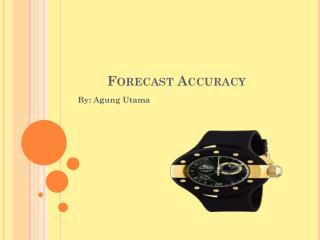DownloadDownload PresentationForecast Accuracy

# Forecast Accuracy

Télécharger la présentation## Forecast Accuracy

- - - - - - - - - - - - - - - - - - - - - - - - - - - E N D - - - - - - - - - - - - - - - - - - - - - - - - - - -
##### Presentation Transcript

1. Forecast Accuracy By: AgungUtama

2. Introduction • A forecast is never completely accurate, there will be always deviation from the actual demand. • This difference between the foracast and the actual is the forecast error. • Although forecast eror is inevitable, the objective of forecasting is that it be as slight as possible. • There are different measures of forecast error, including: Mean Absolute Deviation (MAD), Mean Absolute Percent Deviation (MAPD), Cummulative Error (CE), and Error Bias (E).

3. Mean Absolute Deviation (MAD) • MAD is an average of the difference between the forecast and actual demand, as computed by the following formula: MAD= ΣІDt-Ft I n Where: t =The period number Dt= Demand in period t Ft = The forecast for period t n = The total number of periods I I = Absolute value • The smaller the value of MAD, the more accurate the forecast, although viewed alone, MAD is difficult to assess.

5. Using the data in the table, MAD is computed: • MAD=ΣI Dt-Ft I n = 53l39 11 = 4.85

6. The Mean Absolute Percent Deviation • Measures the absolute error as a percentage of demand rather than per period. • As a result, it eliminates the problem of interpreting the measure of accuracy relative to the magnitude of the demand and forecast values, as MAD does. • A lower percent deviation implies a more accurate forecast. • MAPD =ΣI Dt-Ft I ΣDt = 53.39 520 = 0.10 or 10%

7. Cumulative Error • Cumulative error is computed simply by summing the forecast errors, as shown in the formula: E=Σ et • A large positive value indicates that the forecast is probably consistently lower than the actual demand, or is biased low. • The comulativeeror based on the previuos data is simply computed as: E=Σ et = 49.31

8. Average Error (Bias) • It is computed by averaging the comulative error over the number of time periods • The comulative error is interpreted similarly to the comulative error. A positive value indicates low bias, and a negative value indicates high bias. A value close to zero implies a lack of bias. • The formula is: Ḗ=Σ et n = 49.32 11 = 4.48

9. Discussion questions • Registration number for a marketing seminar over the past 10 weeks are shown below: • Starting with week 2 and ending with week 11, forecast registrations using the naïve forecasting method. • Starting with week 3 and ending with week 11, forecast registrations using a two-week moving average.

10. Demand for heart transplant surgery at RSCM has increased steadily in the past few years: The director of medical services predicted 6 years ago that demand in year 1 would be 41 surgeries. • Use exponential smoothing, first with a smoothing constant of 0.6 and then with 1.9, to develop forecasts for years 2 through 6. • Use MAD criterion, which of the two forecasting methods is best?

11. Given the following data, use least squares regression to derive a trend equation. What is your estimate of the demand in period 7? In period 12?